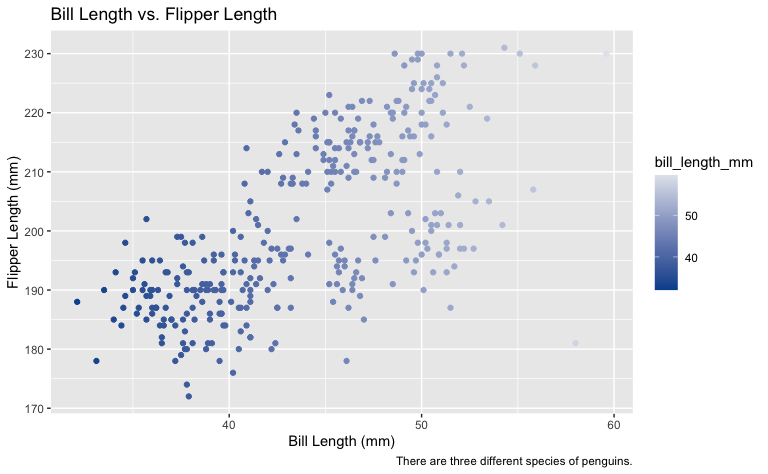# scale_duke_continuous()

This vignette is intended to demonstrate the breadth of scale_duke_continuous() and its various applications. It is fully integrated with ggplot2 visualizations.

# Plot Examples

For these visualizations, we will use the penguins dataset from the palmerpenguins package.

library(duke)
library(palmerpenguins)
library(ggplot2)

## Scatter Plots

plot <- ggplot2::ggplot(palmerpenguins::penguins, ggplot2::aes(bill_length_mm, flipper_length_mm)) +
ggplot2::geom_point(ggplot2::aes(colour = bill_length_mm)) +
ggplot2::labs(title = "Bill Length vs. Flipper Length", caption = "There are three different species of penguins.", x = "Bill Length (mm)", y = "Flipper Length (mm)")

plot +
scale_duke_continuous()